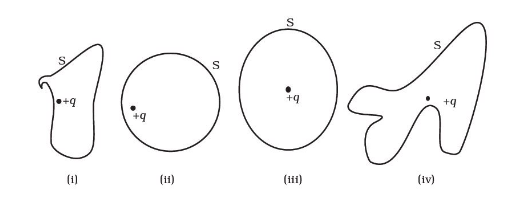# The Electric flux through the surface

Question:

The Electric flux through the surface

(a) in Fig.1.3 (iv) is the largest.

(b) in Fig. 1.3 (iii) is the least.

(c) in Fig. 1.3 (ii) is same as Fig. 1.3 (iii) but is smaller than Fig. 1.3 (iv)

(d) is the same for all the figures.Solution:

The flux of electric field through any closed surface S is 1/ε0 times the total charge enclosed by S (Irrespective of shape and size of the surface).

All the figures given in the questions have the same charge i.e., +q. So, the electric flux through the surface is the same for all the figures.